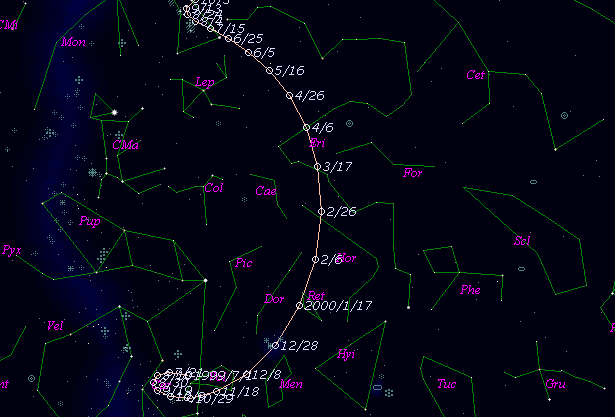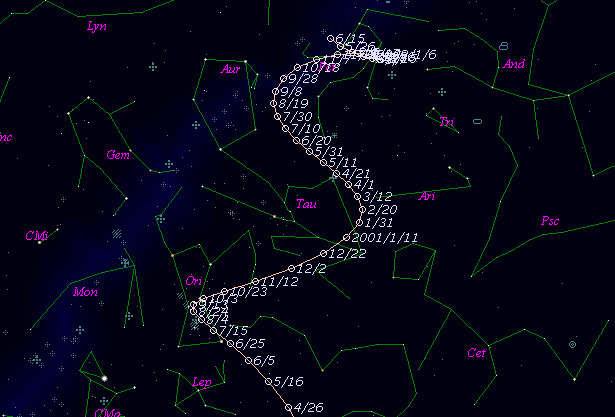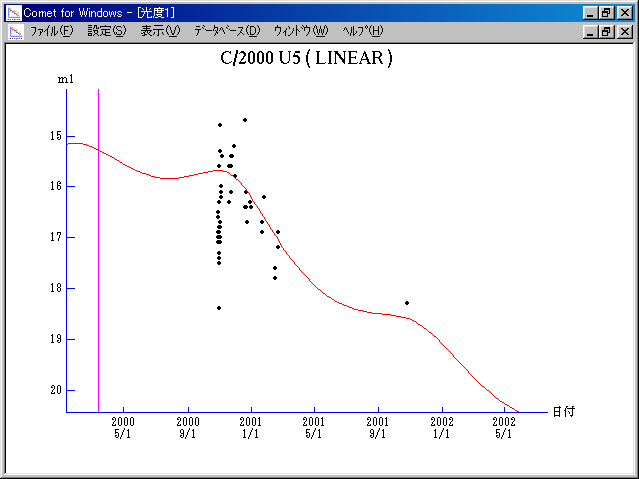# \$B%j%K%"WB@1(B

C/2000 U5 ( LINEAR )

 English version Home page Updated on November 14, 2001###\$B%W%m%U%#!<%k(B

 \$BH/8+F|(B 2000\$BG/(B10\$B7n(B29\$BF|(B \$BH/8+8wEY(B 17.1\$BEy(B \$BH/8+ Lincoln Laboratory Near-Earth Asteroid Research project

###\$B50F;MWAG(B

```   The following improved orbital elements by Kenji Muraoka, are
from 274 observations 2000 Oct. 29 to 2001 Mar. 15, perturbations
by 9 Planets, Moon and 5 minor planets were taken into account.
The mean residual is +/- 0.68 arc seconds.

Epoch  =  2000 Feb. 26.0  TT       JDT = 2451600.5
T  =  2000 Mar. 12.90372       +/- 0.00445 (m.e.) TT
Peri. =  298.92387                +/- 0.00097
Node  =   65.29836                +/- 0.00027   (2000.0)
Incl. =   93.64820                +/- 0.00059
q  =    3.4851831              +/- 0.0000355 AU
e  =    1.0011242              +/- 0.0000514
1/a  =   -0.0003226              +/- 0.0000147 1/AU
orig. 1/a  =   +0.0003569
fut.  1/a  =   -0.0016624
```

###\$B@1?^(B###\$B8wEYJQ2=(B

```        m1 = 7.0 + 5 log\$B&\$(B + 10.0 log r
```##### \$B50F;MWAG\$OB<2,7r<#;a\$N7W;;\$K\$h\$k\$b\$N\$G\$9!#(B \$B@1?^\$O(B StellaNavigator Ver.2.0 for Windows (\$B%"%9%H%m%"!<%D(B \$BJTCx(B / \$B%"%9%-!<=PHG6I4)(B) \$B\$G:n@.\$7\$?\$b\$N\$G\$9!#(B \$B8wEY%0%i%U\$O(BComet for Windows\$B\$G:n@.\$7\$?\$b\$N\$G\$9!#(B